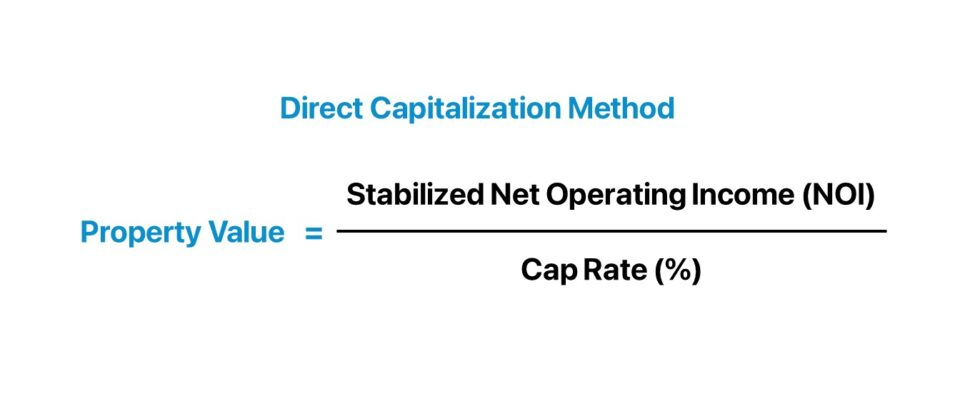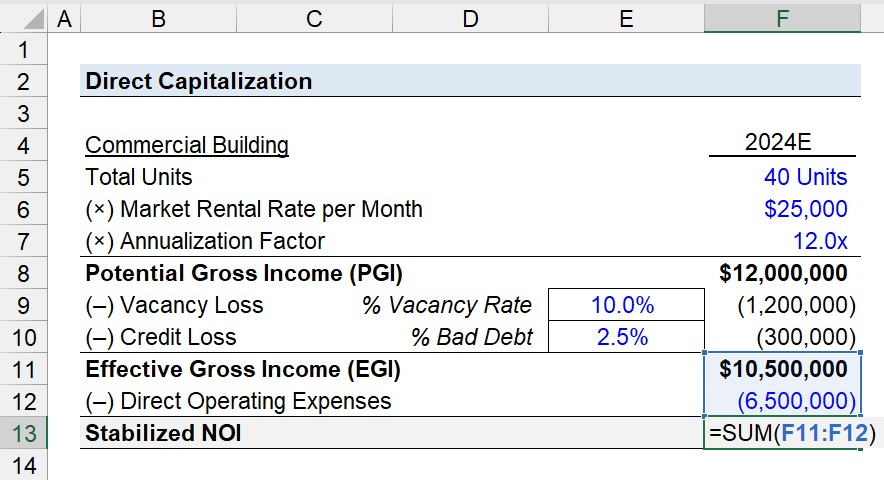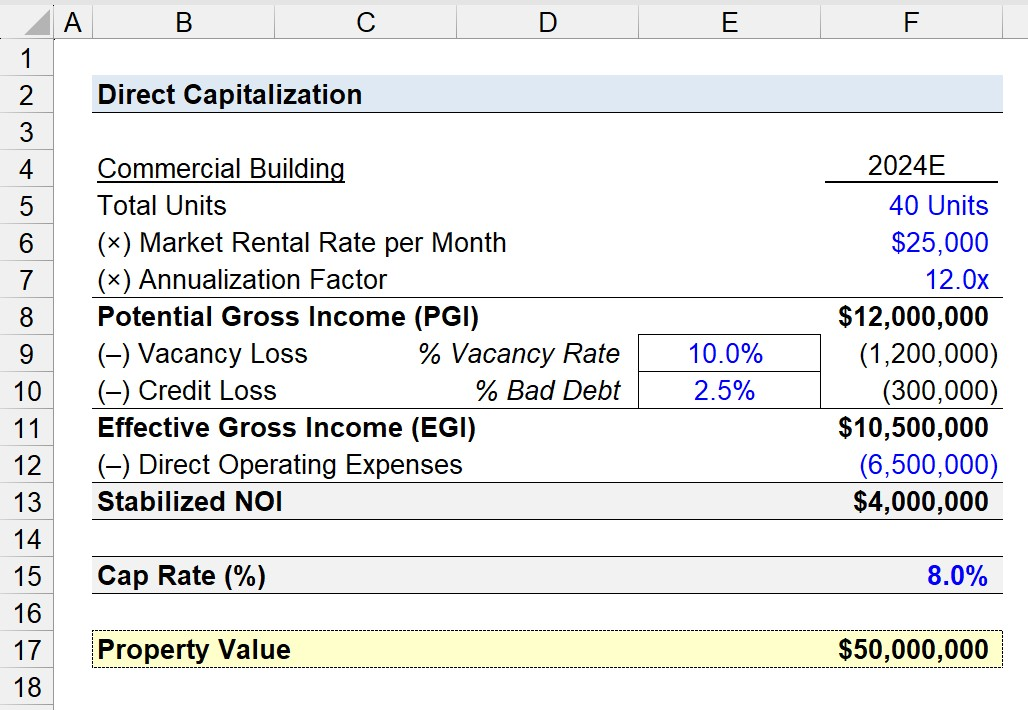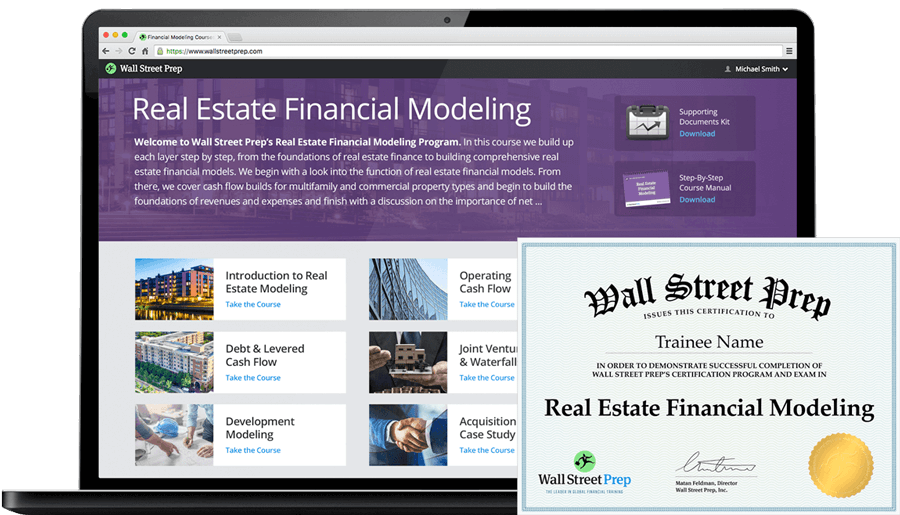Welcome to Wall Street Prep! Use code at checkout for 15% off.# Direct Capitalization

Guide to Understanding the Direct Capitalization Method in Real Estate## How to Calculate Direct Capitalization?

The direct capitalization method is a standard real estate appraisal method frequently used among industry practitioners to estimate the value of a property.

Under the direct capitalization method – one of the two approaches categorized under the “Income Approach” designation – the implied property value is determined by dividing the net operating income (NOI) of a given property by an appropriate cap rate based on market data on comparable properties.

• Net Operating Income (NOI) → The NOI of a property equals the sum of its projected rental income and ancillary income, minus direct operating expenses. The greater the NOI of a property, the higher the property value (and vice versa).
• Cap Rate (%) → The cap rate, shorthand for “capitalization rate,” is the expected return on an income-generating property investment. As the cap rate decreases, the value of the property increases (and vice versa).

By dividing the net operating income (NOI) of a property – most often projected on a twelve-month basis (or until the property has reached stabilization) – by an appropriate cap rate, the projected income stream in one year is converted into an estimate of the property’s value.

Therefore, the direct capitalization method determines the valuation of income-generating, rental properties based on their potential to generate NOI and the market cap rate.

The stabilized NOI and cap rate are each pro-forma measures determined by analyzing the relevant market data on comparable properties around factors such as the current rental rates (i.e. pricing), vacancy rates (or the inverse, occupancy rates), credit loss rates around the collection of rental payments from tenants, and the incurred operating expenses (Opex).

The step-by-step process to calculate the valuation of a property is as follows.

1. Calculate Net Operating Income (NOI) → The initial step is to project the stabilized NOI of the property, where the key operating drivers are the vacancy and credit loss rates, as well as the operating expense assumptions.
2. Determine Cap Rate → The cap rate assumption is determined by compiling market research and analyzing the data on comparable properties currently on sale or was part of a recent transaction to establish parameters to guide pricing.
3. Estimate Property Value (NOI / Cap Rate) → In the final step, the property value can be estimated under the income approach by dividing the stabilized NOI of the property by an appropriate cap rate.## Direct Capitalization Formula

The formula to perform a real estate appraisal under the direct capitalization method divides a property’s NOI by the market cap rate (%).

Property Value = Net Operating Income (NOI) ÷ Cap Rate (%)

Where:

• Net Operating Income (NOI) = (Rental Income + Ancillary Income) – Direct Operating Expenses
• Cap Rate (%) = Net Operating Income (NOI) + Property Value

Conversely, the net operating income (NOI) can be expressed using the following formula.

Where:

Generally speaking, the direct capitalization method is most reliable for stabilized, income-generating properties, where there is more than sufficient market data to support the validity of the cap rate assumption.

The drawback to the direct capitalization method is the implicit assumption that NOI (and operating costs) will remain constant, i.e. the stabilized NOI reflects the income of the property going forward.

With that said, the direct capitalization method is thus most suitable for properties that generate consistent income, where the projected NOI at stabilization is not too far-fetched (and is backed by historical data).

## Direct Capitalization Calculator

We’ll now move to a modeling exercise, which you can access by filling out the form below.#### Excel TemplateSubmitting...

## 1. Commercial Real Estate (CRE) Property Assumptions

Suppose a real estate investor is tasked with performing an appraisal on the valuation of a commercial property in consideration to be a potential strategic acquisition.

The property, a commercial building, is currently undergoing minor construction and repair work, which is expected to be finalized by 2024.

The commercial building has a total of 40 units and the market rate rent is \$25k per month.

Once the commercial building is stabilized in 2024, the pro forma projections are as follows.

2024E Projections

• Total Number of Units Available for Rent = 40 Units
• Market Rental Rate per Month = \$25,000
• Annualization Factor = 12.0x
• Vacancy Loss (% of PGI) = 10.0%
• Credit Loss (% of PGI) = 2.5%

The first step is to calculate the commercial building’s potential gross income (PGI) by multiplying the total number of units available for rent by the rent charged per month.

The product of the units available for rent and monthly rental pricing must then be converted into an annualized PGI, which comes out to be \$12 million.

• Potential Gross Income (PGI) = 40 Units × \$25,000 × 12 = \$12 million

From the potential gross income (PGI) metric, the subsequent step is to deduct vacancy and credit losses – which is an inevitable part of the leasing business model – to compute the effective gross income (EGI) metric, which amounts to \$4.2 million.

• Vacancy Loss = 10.0% × \$12 million = \$1.2 million
• Credit Loss = 2.5% × \$12 million = \$300k
• Effective Gross Income (EGI) = \$12 million – \$1.2 million – \$300k = \$10.5 million

## 2. Stabilized NOI Calculation Example

In the next section, we’ll estimate the stabilized NOI of the commercial building by subtracting direct operating expenses from the effective gross income (EGI) of \$10.5 million.

As for our assumption on the property’s total operating expense, we’ll assume the annual Opex incurred per year is projected to be about \$6.5 million.

• Direct Operating Expenses = \$6.5 million

Since the two necessary inputs to determine the stabilized NOI have been determined, the remaining step is to subtract direct operating expenses from the effective gross income (EGI) to arrive at a stabilized NOI of \$4 million.

• Stabilized NOI = \$10.5 million – \$6.5 million = \$4 million## 3. Direct Capitalization Calculation Example

In the final part of our real estate valuation tutorial, we’ll estimate the property value of our hypothetical commercial building using the direct capitalization method.

The only assumption left is the cap rate, which we’ll assume is 8.0%, based on the analysis performed on the observable market data of comparable properties.

• Cap Rate (%) = 8.0%

Given the cap rate, the denominator in the property value formula, we’ll divide the stabilized NOI of \$4 million by 8.0% to arrive at an implied property value of \$50 million.

• Property Value = \$4 million ÷ 8.0% = \$50 million20+ Hours of Online Video Training

### Master Real Estate Financial Modeling

This program breaks down everything you need to build and interpret real estate finance models. Used at the world's leading real estate private equity firms and academic institutions.

Inline Feedbacks